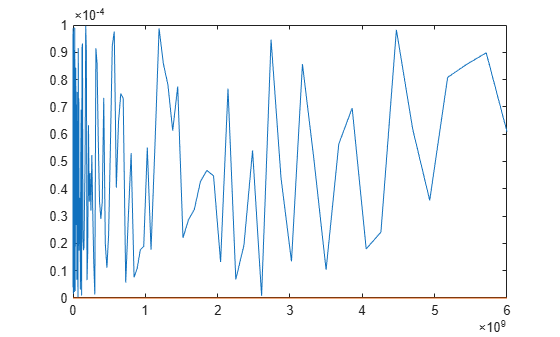rational

Perform rational fitting to complex frequency-dependent data

Description

Use the rational object and an interpolative algorithm to create a rational fit to frequency-dependent data.

The complex frequencies are given by the equation:

Creation

Description

fit = rational(freq,data) returns a rational object with complex frequencies using the given frequency vector and network parameter data.

example

fit = rational(s) returns a rational object for N-port S-parameters.

fit = rational(___,tol) returns a rational object fit that satisfies a relative error tolerance. Specify tol after any of the input argument combinations from the previous syntaxes.

[fit,error] = rational(___) also returns the error of the fit. Use any of input argument combinations from the previous syntaxes.

example

fit = rational(___,Name,Value) sets properties using one or more name-value arguments. For example, fit = rational(s,'Tolerance',-34)sets the relative error tolerance in decibels for the fit. Specify name-value arguments after any of the input arguments from the previous syntaxes.

Input Arguments

expand all

Nonnegative frequencies, specified as a vector of nonnegative frequencies in Hz.

Data Types: double

Network parameter data, specified as a vector, a 2-D array or a 3-D array. The length of the data values must equal the length of the frequency values.

Relative error tolerance, specified as a scalar less than or equal to zero. tol value sets the input for the 'Tolerance' property.

Data Types: double

N-port S-parameters, specified as an N-by-N matrix of elements of S sharing identical poles.

Properties

expand all

Relative error tolerance, specified as a scalar less than or equal to zero.

Data Types: double

Behavior of fit for large S-parameters, specified as true or false. When true, the direct term in the fit is set to zero so that the rational fit F(S) tends to zero as S approaches infinity. When false, a nonzero direct term is allowed.

Data Types: logical

Maximum number of poles, specified as a scalar nonnegative integer.

Data Types: double

Error metrics in rational object, specified as one of the following:

• If you specify 'ErrorMetric' as 'default', the rational object distributes the error evenly.

• If you specify 'ErrorMetric' as 'Relative', the rational object fits both peaks and valleys or gets smaller error for smaller values.

Data Types: char

Ignores low-level noise in data, specified as a scalar.

Example: NoiseFloor = -60

Data Types: double

Pole stability, specified as a logical true (1) or false (0). When you specify true, all the poles of the fit are stable. When you specify false,the poles can be anywhere in the complex plane.

Example: Casual = false

Maximum value of the quality factor of the poles of the fit, specified as a positive scalar.

Example: Qlimit = 1100

Data Types: double

Data reduction, specified as a logical 1 (true) or 0 (false). When you specify true, the function reduces the data for the fitter to save memory and computation time. Reduce data for the fitter in order to save memory and computation time, specified as a logical true or false.

Example: ColumnReduce = false

Display options for the fitting algorithm of the rational object, specified as one of the following:

• 'off'— No display

• 'on'— Printed information

• 'plot'— Plots of the interpolation progress

• 'both'— Both printed information and plots.

Data Types: char

Object Functions

 timeresp Time response for rational object and rationalfit function object stepresp Step-signal response for rational object and rationalfit function object freqresp Frequency response of rational object and rationalfit function object pwlresp Calculate time response of piecewise linear input signal impulse Impulse response for rational function object ispassive Return true if rationalfit output is passive at all frequencies makepassive Enforce passivity of rationalfit output or a rational object passivity Plot passivity of N-by-N rationalfit function output generateSPICE Generate SPICE file from rationalfit of S-parameters

Examples

collapse all

Create S-Parameters from the file named passive.s2p.

S = sparameters('passive.s2p');

Perform rational fitting of the S-parameters.

fit = rational(S);

Create an S-Parameters object from the file named default.s2p. Perform rational fitting of the S-Parameters.

S = sparameters('default.s2p');
fit = rational(S,'Display', 'plot')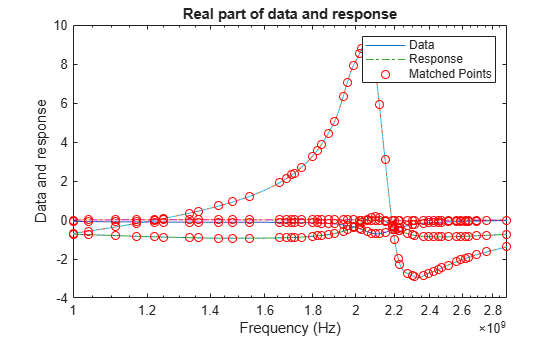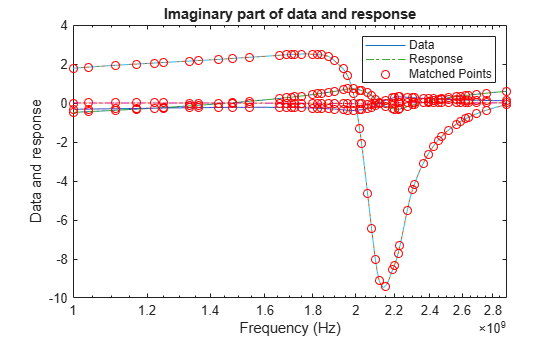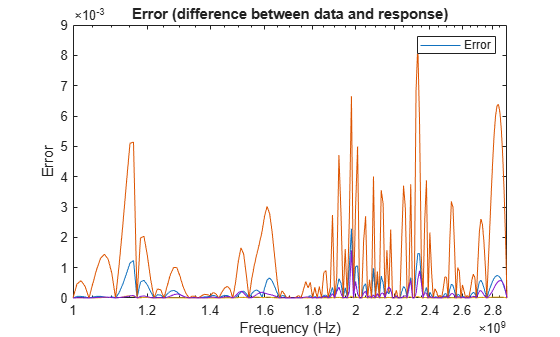fit =
rational with properties:

NumPorts: 2
NumPoles: 44
Poles: [44x1 double]
Residues: [2x2x44 double]
DirectTerm: [2x2 double]
ErrDB: -22.6464

Calculate the zeros, poles, gain, and DC gain of the rational object.

[z,p,k,dcgain] = zpk(fit)
z=2×2 cell array
{43x1 double}    {43x1 double}
{43x1 double}    {43x1 double}

p=2×2 cell array
{44x1 double}    {44x1 double}
{44x1 double}    {44x1 double}

k = 2×2
109 ×

-2.5743   -0.0556
-0.6980   -1.3221

dcgain = 2×2

0.0933   -0.0302
-1.1079    0.4746

Create an S-parameters object from the specified S2P file.

S = sparameters('sawfilterpassive.s2p');
f = S.Frequencies;

Create a rational object with the tolerance of -40 dB.

fit = rational(S,-40);

Compare the fit to the data. You can see the deviations at the smaller values on a semi-log plot.

dresp = freqresp(fit,f);
plot(f,20*log10(abs(squeeze(S.Parameters(2,1,:)))),...
f,20*log10(abs(squeeze(dresp(2,1,:)))),f,20*log10(abs(squeeze(S.Parameters(2,1,:)-dresp(2,1,:)))))
title('Default Fitting for sawfilterpassive.s2p');
ylabel('dB');
xlabel('Frequency (Hz)');
legend('Data','Fit','Error','Location','northwest');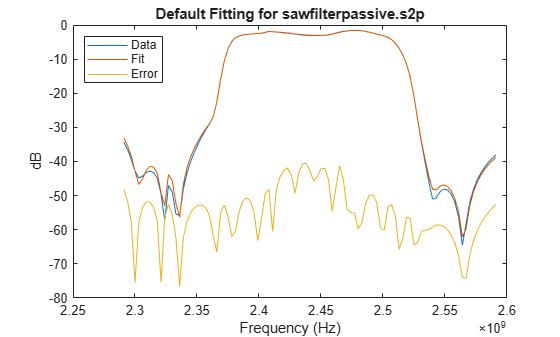Create a rational object with 'ErrorMetric' set to 'Relative' to fit both peaks and valleys.

rfit = rational(S,-40,'ErrorMetric','Relative');

Compare the fit to the data. The peaks and valleys are fitted.

rresp = freqresp(rfit,f);
figure(2)
plot(f,20*log10(abs(squeeze(S.Parameters(2,1,:)))),...
f,20*log10(abs(squeeze(rresp(2,1,:)))), f, 20*log10(abs(squeeze(S.Parameters(2,1,:)-rresp(2,1,:)))));
title('Relative Error Fitting for sawfilterpassive.s2p');
ylabel('dB');
xlabel('Frequency (Hz)');
legend('Data','Fit','Error','Location','northwest');Create an S-parameters object from the specified S2P file.

S = sparameters('passive.s2p');
f = S.Frequencies;
data = S.Parameters;

Set one of the data entries to zero.

data(2,2,:) = 0;

Create a rational object with the tolerance of -40 dB.

fit = rational(f,data,-40);

Compare the fit to the data. The fit and the data match closely.

xresp = freqresp(fit,f);
figure(3)
plot(f,abs(squeeze(data(2,1,:))),f,abs(squeeze(xresp(2,1,:))))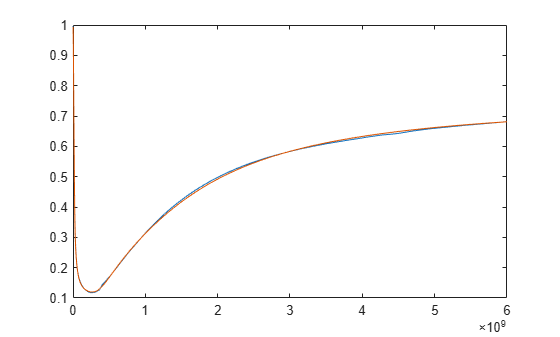Add noise to the data and create a rational object.

rng(1);
noisyData = data + 1e-4 * rand(size(S.Parameters));
nfit = rational(f,noisyData,-40);

Compare the fit to the data with noise. Noise cannot be fitted because this is a data with a higher order fit with a worse error metric.

nresp = freqresp(nfit,f);
figure(4)
plot(f,abs(squeeze(noisyData(2,2,:))),f,abs(squeeze(nresp(2,2,:))))Create a rational object with the noise floor of -60 dB and plot the fit. The fitter ignores low-level noise.

ffit = rational(f,noisyData,-40,'NoiseFloor',-60);
fresp = freqresp(ffit,f);
figure(5)
plot(f,abs(squeeze(noisyData(2,2,:))),f,abs(squeeze(fresp(2,2,:))))